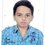# For all IMO 2016 aspirants

Hello INMO, USAMO and all national olympiads awardees, try these problems and post solutions , specially for IMO 2016:

$(1)$ Find all functions $f, g : R \rightarrow R$ which satisfy the equation:

$(x - y)f(z) + (y - z)f(x) + (z - x)f(y) = g(x + y + z)$, for all real numbers $x, y, z$ such that $x \neq y, y \neq z, z \neq x$.

$(2)$ Show that if $p$ is a prime and $0 \le m < n < p$, then

$\huge\ \left( \begin{matrix} np+m \\ mp+n \end{matrix} \right) \equiv { \left( -1 \right) }^{ m+n+1 }p \pmod{{ p }^{ 2 }}$.

$(3)$ Given two circles that intersect at $X$ and $Y$, prove that there exist four points with the following property. For any circle $\wp$ tangent to the two given circles, we let $A$ and $B$ be the points of the tangency an $C$ and $D$ the intersections of $\wp$ with the line $XY$. Then each of the lines $AC, AD, BC, BD$ passes through one of these four points.

$(4)$ Find all integral solutions to following equations:

$(a)$ $\huge\ { y }^{ { y }^{ { y }^{ y } } } + { x }^{ { x }^{ x } } = { \left( x!.y! \right) }^{ 2016 }$,

$(b)$ $\huge\ { a }^{ x } + { b }^{ { y }^{ z } } + { c }^{ { z }^{ { x }^{ d } } } + { d }^{ { a }^{ { y }^{ { z }^{ x } } } } = { \left( abcd \right) }^{ xyz }$.

$(5)$ Let $a$, $b$, $c$ be positive real numbers. Prove that

$\large\ { \left( \sum { \frac { a }{ b+c } } \right) }^{ 2016 } \le \left( \sum { \frac { { a }^{ 2016 } }{ { b }^{ 2016 } + { b }^{ 2015 }c } } \right) { \left( \sum { \frac { a }{ c + a } } \right) }^{ 2015 }$.

$(6)$ Let $I$ be the in-center of triangle $ABC$. It is known that for every point $M \in (AB)$, one can find the points $N \in (BC)$ and $P \in (AC)$ such that $I$ is the centroid of the triangle $MNP$. Prove that $ABC$ is an equilateral triangle.Note by Priyanshu Mishra
5 years, 6 months ago

This discussion board is a place to discuss our Daily Challenges and the math and science related to those challenges. Explanations are more than just a solution — they should explain the steps and thinking strategies that you used to obtain the solution. Comments should further the discussion of math and science.

When posting on Brilliant:

• Use the emojis to react to an explanation, whether you're congratulating a job well done , or just really confused .
• Ask specific questions about the challenge or the steps in somebody's explanation. Well-posed questions can add a lot to the discussion, but posting "I don't understand!" doesn't help anyone.
• Try to contribute something new to the discussion, whether it is an extension, generalization or other idea related to the challenge.

MarkdownAppears as
*italics* or _italics_ italics
**bold** or __bold__ bold
- bulleted- list
• bulleted
• list
1. numbered2. list
1. numbered
2. list
Note: you must add a full line of space before and after lists for them to show up correctly
paragraph 1paragraph 2

paragraph 1

paragraph 2

[example link](https://brilliant.org)example link
> This is a quote
This is a quote
    # I indented these lines
# 4 spaces, and now they show
# up as a code block.

print "hello world"
# I indented these lines
# 4 spaces, and now they show
# up as a code block.

print "hello world"
MathAppears as
Remember to wrap math in $$ ... $$ or $ ... $ to ensure proper formatting.
2 \times 3 $2 \times 3$
2^{34} $2^{34}$
a_{i-1} $a_{i-1}$
\frac{2}{3} $\frac{2}{3}$
\sqrt{2} $\sqrt{2}$
\sum_{i=1}^3 $\sum_{i=1}^3$
\sin \theta $\sin \theta$
\boxed{123} $\boxed{123}$

Sort by:

I dunno hows this

- 5 years, 5 months ago

Please explain clearly what you want to say.

- 5 years, 5 months ago

any hints to the last question?

- 4 years, 6 months ago